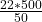## 4 points Write the sentence as a proportion. Then solve the proportion. 22 is to 50 as x is to 500

Question

4 points
Write the sentence as a proportion. Then solve the proportion. 22 is to 50
as x is to 500

in progress 0
6 months 2021-07-29T02:07:48+00:00 2 Answers 5 views 0

x = 220

Step-by-step explanation:

22/50 = x/500

50x = 22 * 500

Divide both sides by 50.

x = 22 * 10

x = 220

220

Step-by-step explanation:

22 : 50 = x : 500

Product of means = product of extremes

50 *x = 22 *500

x =x = 22 * 10

x = 220